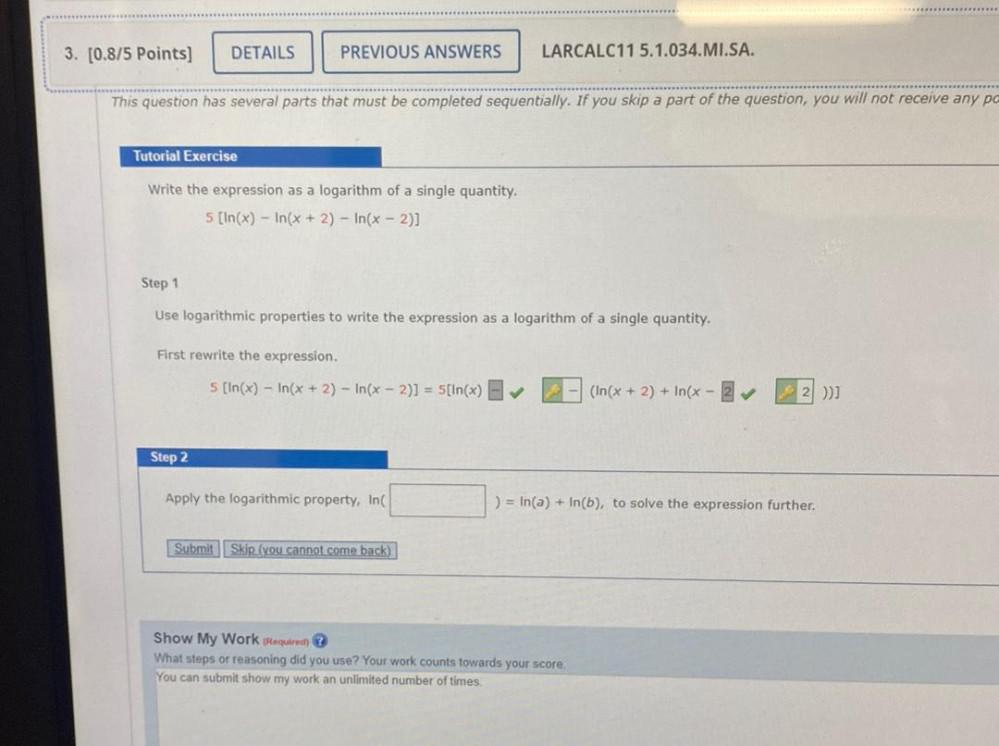Question:

# 3. (0.875 Points] DETAILS PREVIOUS ANSWERS LARCALC115.1.034.MI.SA. This question has several parts that must be completed sequen3. (0.875 Points] DETAILS PREVIOUS ANSWERS LARCALC115.1.034.MI.SA. This question has several parts that must be completed sequentially. If you skip a part of the question, you will not receive any po Tutorial Exercise Write the expression as a logarithm of a single quantity. 5 [In(x) - In(x + 2) - In(x - 2)] Step 1 Use logarithmic properties to write the expression as a logarithm of a single quantity, First rewrite the expression. 5 [In(x) - In(x + 2) - In(x - 2)] = 5[ln(x) (In(x + 2) + In(x - -22) Step 2 Apply the logarithmic property. In ) = In(a) + In(b), to solve the expression further. Submit Skip (you cannot come back) Show My Work Required What steps or reasoning did you use? Your work counts towards your score. You can subrnit show my work an unlimited number of times.